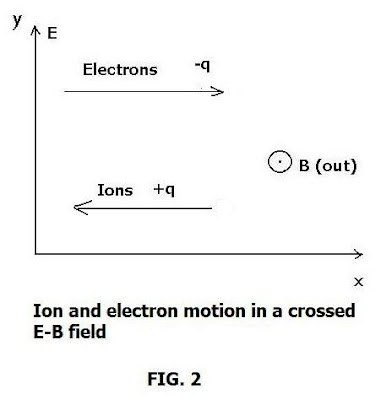## Wednesday, February 9, 2011

### Particle Orbital Dynamics in the MagnetosphereThe magnetosphere holds special importance for planet Earth as the primary boundary protecting us from the harshest solar radiation as well as charged particles. Part of the dynamic for doing this entails the actual trapping of charged particles, for example in magnetic mirror systems that operate within the magnetosphere- assuming specific properties.

In this and two future blogs, I'll examine the particle dynamics closely especially as they tend to the limit of brief but defined orbits. We find that at some stage these become chaotic and chaotic or non-linear dynamics must be used to predict charged particle behavior. There is even the possibility of particle escape from its magnetic or other confines.

In this process, I'll examine the orbits of charged particles (electron, protons) of Ek = 1.0 MeV analyzed through multiple bounce periods t(b). This will include varying L-parameters (5 less than or equal to, L less than or equal to 1.5) in a magnetospheric mirror (particle trap) system, such that a first order Fermi acceleration governs the dynamics.

Two modes of analyses are performed: I) Normal time step sequence: Computing the respective orbits over n t (b) bounce periods, for 1 MeV electrons and protons trapped between the mirrors (initialized at position, M1-M2 = L(10)) and, II) Chaotic time sequence imposed via Poincare mapping time step. In this regime, the time step in (I) is adjusted to reflect an applied force (Fermi force) acting once per mirror-cycle (bounce period) such that:

t(e) n = nt(e) b + 2πn/ W(e) and t(p) n = nt(p) b + 2πn/ W(p)

Where W(e) , W(p) are the electron and proton gyro-frequencies.

Introduction.

The Earth’s near magnetosphere represents an ongoing, fruitful environment for the study of particle dynamics and chaos, governed by changing magnetic inductions, velocities and pitch angle variations. By “near magnetosphere” is meant the region extending from the ionosphere to a distance of 2 RE, where 1 RE = one Earth radius. It includes the auroral oval, the magnetopause (to 2 RE ); the plasmasphere, plasma sheet, polar cusp and ionosphere. (See graphic)

Here we enter plasma “orbit theory” and consider a charged particle (say of charge q) in a uniform and constant magnetic field (B).

The governing equation of motion is:

(1) m (dv/dt) = q(v X B)

The motion here is such that v is always perpendicular to the force acting on the particle so:
v ⊥ F, implying circular motion.

Thus:

(2) dv/dt = q/ m [v X B] is a centripetal acceleration.

e.g. a = (-r) (v⊥)^2/ r

Note that the velocity has two components:

(3) v = v⊥ + v ‖

where the second term denotes the velocity along B (magnetic induction) which stays constant so that

(4) dv ‖ / dt = 0

Meanwhile:

(5) (v⊥ )^2/ r = q/ m [v⊥ B]

the quantity r is none other than the gyro-radius. Solving for it one finds:

(6) r = m/ q [v⊥ / B] = v⊥ / (qB/m)

for which one can have either the electron:

(7a) W(e) = qB/m_e

or the ion, gyrofrequency:

(7a) W(i) = qB/m_i

As shown in Fig. 2, both electrons and ions (e.g. protons) undergo a corkscrew motion around the centers of their paths (guiding centers) and have drift velocities in the same direction in this field orientation.

This drift motion occurs in crossed (E X B) fields and hence this is called “E cross B” drift. In the magnetosphere, special focus is on the motion of charged (e, i) particles undergoing periodic motion between two mirror points.

In space physics, one uses the sine of the loss cone angle to obtain the mirror ratio (where
B(min) , B (max) refer to minimum and maximum magnetic induction respectively):

sin(Θ_L) = ± [ (B(min) / B (max)]^½

If one finds that there are particles within the “mirrors” for which the “pitch angle” (φ) has:

(9) sin (φ) > [ (B(min) / B (max )]^½

then these will be reflected within the tube, On the other hand, those particles for which the “less than” condition applies will be lost, on transmission out of the mirror configuration.

Since the adiabatic invariant for particle motion is a constant of the motion:

(10) u= ½ [mv ^2 /B]

we have:

(11) [v⊥^2 /B(max)] = [v ‖ ^ 2 /B_z] = const.
or [v⊥^2 /v ‖ ^ 2 ] = B_z /B(max)

where we take B_z = B(min)

For kinetic energy of particles we must have:

(12) E = ½ m (v⊥^2 + v ‖ ^ 2 ) = const.

So that E is a constant of the motion, as well as u. Both of these are called “adiabatic invariants”.

Part 2: The Third Adiabatic Invariant (to come)